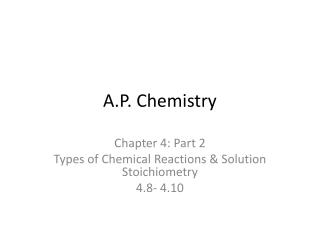DownloadDownload PresentationA.P. Chemistry

# A.P. Chemistry

Télécharger la présentation## A.P. Chemistry

- - - - - - - - - - - - - - - - - - - - - - - - - - - E N D - - - - - - - - - - - - - - - - - - - - - - - - - - -
##### Presentation Transcript

1. A.P. Chemistry Chapter 4: Part 2 Types of Chemical Reactions & Solution Stoichiometry 4.8- 4.10

2. 4.8 Acid-Base Aqueous Reactions (p. 157) Definition of Acid- substances that ionize in water to produce hydrogen ions Definition of Base- substances that ionize in water to produce hydroxide ions AcidsBases Sour taste bitter taste Cause color changes in plant dyes feel slippery React w/ certain metals (Zn, Mg, Fe), cause color changes in plant dyes Producing hydrogen gas aqueous solns conduct electricity React w/ carbonates & bicarbonates, Producing carbon dioxide gas Aqueous solns conduct electricity Strong AcidsWeak Acids HCl, HBr, HI, HNO3, HF, HNO2, H3PO4, HC2H3O2, organic acids H2SO4, HClO4 Strong BasesWeak Bases NaOH, KOH NH3

3. Neutralization Reactions- a reaction between an acid and a base, generally producing a salt and water Acid + base  salt + water HCl(aq) + NaOH(aq) NaCl (aq) + H2O(l) H+(aq) + OH-(aq)  H2O(l) net ionic

4. Calculations using Titration Data: M1V1 = M2V2 M = mol/L Volume in liters (be sure to convert mL L) (p. 145-147) Problem: What volume of 16 M sulfuric acid must be used to prepare 1.5 L of a 0.10 M H2SO4 solution?

5. Titration- a solution of accurately known concentration, called a standard solution, is added gradually to another solution of unknown concentration, until the chemical reaction between the two solutions is complete. The equivalence point or stoichiometric point is the point at which the acid has completely reacted with or been neutralized by the base. Indicators are substances that have distinctly different colors in acid or base media and are used to determine the equivalence point. The point at which the indicator changes color is the endpoint. Example: What volume of a 0.100 M HCl solution is needed to neutralize 25.0 mL of 0.350 M NaOH? Example: in a titration experiment, a student finds that 35.18 mL of a KOH solution are needed to neutralize 0.5468 of potassium acid phthalate (KHP), KHC8H4O4, a monoprotic acid. What is the molarity of the KOH?

6. 4.9 Oxidation- Reduction Reactions Oxidation-Reduction Aqueous Chemistry- electron-transfer reactions (p. 164) Definition of Oxidation- loss of electrons Definition of Reduction- gain of electrons

7. DeterminingOxidation Number (p. 167) free elements have an oxidation state of 0 monatomic ions: oxidation state is equal to the charge oxygen: is –2 in most compounds; -1 in hydrogen peroxides or peroxide ion hydrogen: is +1 , except when it is bonded with a metal in a binary compound; hydride ion is –1 fluorine: -1 in all compounds other halogens: -1 when monatomic ions, positive oxidative states in oxyanions sum of oxidation numbers in a neutral molecule = 0 sum of oxidation numbers in a polyatomic ion must equal the charge on the ion (rare) oxidation numbers need not be integers, for ex., Oxygen in O2-1 is –1/2

8. Example: Assign oxidation states to all atoms in the following: a. CO2b. SF6c. NO3-1

9. Writing Half-Reactions- a Half-reaction explicitly shows the electrons being lost or gained by the different chemical species in a chemical reaction; the sum of the half-reactions gives the overall reaction. Electrons are the product in the oxidation half-reaction; they are the reactant in a reduction half-reaction. (p. 171) 2Na(s) + Cl2(g)  2NaCl(s) oxidation: 2 Na  2Na+1 + 2e- reduction: Cl2 + 2e-  2Cl-1 Example: Write the half-reactions for the following reaction: 2Al(s) + 3I2(s)  2AlI3(s)

10. Different Types of REDOX Reactions (p. 164) Combination (synthesis), decomposition (analysis), combustion, and single replacement reactions are all types of Redox reactions. There is also a reaction type called disproportionation in which an element in one oxidative state is simultaneously oxidized and reduced.(p. 950) Double replacement reactions, acid-base neutralization reactions, and precipitation reactions do not involve changes in oxidative state, and therefore, will not be redox reactions. Predicting Displacement Reactions Example: Which of these reactions will not occur? Al + CuCl2 Cu + AlCl3 Cl2 + NaBr Br2 + NaCl

11. 4.10 Balancing Oxidation- Reduction Equations Balancing Redox Reactions – In Acid Solution • Write the ½ reactions • Balance all elements except for H & O • Balance O, using H2O • Balance H, using H+ • Balance charges, using e- • Balance # of electrons in the 2 reactions • Add the two ½ reactions, cancel like terms • Check that all species are balanced

12. In Base Solution (p. 176-177) • Do all of the steps above (for Acid Solution) • Add OH- to both sides of the equation equal to the # of H+ (this forms water) • Cancel H2O that appears on both sides of equation • Check that all species are balanced

13. (NOTE: the example on p. 137-8 of your textbook is the redox reaction in the Redox Titration Lab and was a question on the 2007 AP Chemistry exam.) Redox Titration- when a solution containing an oxidizing agent is titrated with a solution containing a reducing agent. The equivalence point is reached when the reducing agent is completely oxidized by the oxidizing agent. Two common oxidizing agents are KMnO4 and K2Cr2O7; the colors of the reduced species is distinctly different from the polyatomic ions. Example: A 16.42 mL volume of 0.1327 M KMnO4 solution is needed to oxidize 25.00 mL of a FeSO4 solution in an acidic medium. Determine the molarity of the FeSO4 solution.